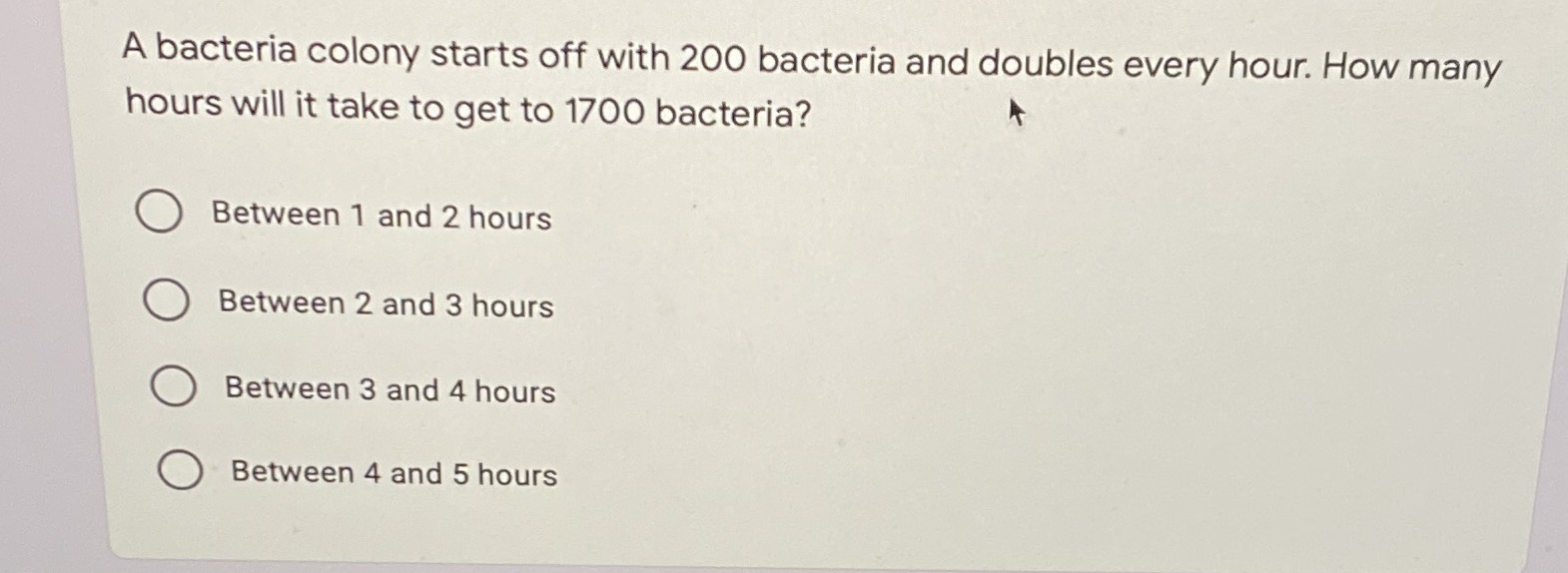### ¿Todavía tienes preguntas de matemáticas?

Pregunte a nuestros tutores expertos
Algebra
PreguntaA bacteria colony starts off with $$200$$ bacteria and doubles every hour. How many  hours will it take to get to $$1700$$ bacteria?

A. Between $$1$$ and $$2$$ hours

B. Between $$2$$ and $$3$$ hours

C. Between $$3$$ and $$4$$hours

D. Between $$4$$ and $$5$$ hours